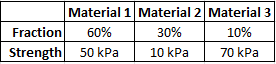## Composite Strain

If a composite material has the known characteristics shown, and a modulus of elasticity of 500 kPa, what is its strain?Hint
$$\sigma_c=\sum f_i\sigma_i$$$where $$\sigma_c$$ is the strength parallel to the fiber direction, $$f_i$$ is the volume fraction of the individual material, and $$\sigma_i$$ is the individual material’s strength. Hint 2 Hooke’s Law: $$\sigma=E\varepsilon$$$
where $$\sigma$$ is the stress, $$E$$ is the elastic modulus (modulus of elasticity or Young’s modulus), and $$\varepsilon$$ is the strain.
A composite material is a material formed from two or more “base” materials. The base/basic materials have notably dissimilar physical or chemical properties, but when merged to create a composite, produce properties unlike the individual elements. To find a composite’s strength:
$$\sigma_c=\sum f_i\sigma_i$$$where $$\sigma_c$$ is the strength parallel to the fiber direction, $$f_i$$ is the volume fraction of the individual material, and $$\sigma_i$$ is the individual material’s strength. Thus, $$\sigma_c=f_1 \sigma_1+f_2\sigma_2+f_3\sigma_3$$$
$$=(0.6)(50kPa)+(0.3)(10kPa)+(0.1)(70kPa)$$$$$=30kPa+3kPa+7kPa=40\:kPa$$$
Hooke’s Law:
$$\sigma=E\varepsilon$$$where $$\sigma$$ is the stress, $$E$$ is the elastic modulus (modulus of elasticity or Young’s modulus), and $$\varepsilon$$ is the strain. Therefore, $$\varepsilon=\frac{\sigma_c}{E}=\frac{40kPa}{500kPa}=0.08$$$
0.08